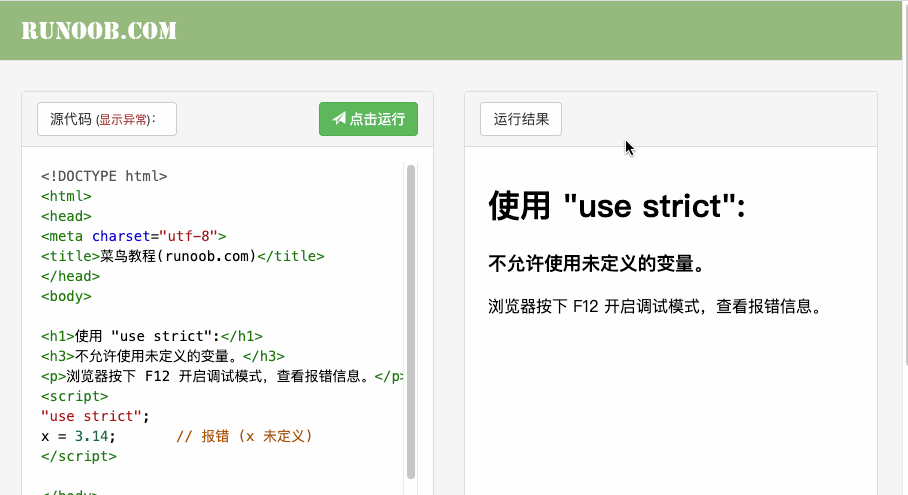﻿ JavaScript 严格模式(use strict) | 开发测试教程
F:\personal\devqa\htmlfiles\dev_reviewed\js\partial_content\js-use-strict.html

# JavaScript 严格模式(use strict)

JavaScript 严格模式（strict mode）即在严格的条件下运行。

## 使用 "use strict" 指令

"use strict" 指令在 JavaScript 1.8.5 (ECMAScript5) 中新增。

"use strict" 的目的是指定代码在严格条件下执行。

Internet Explorer 10 +、 Firefox 4+
Chrome 13+、 Safari 5.1+、 Opera 12+。

## 严格模式声明### 实例

"use strict";x = 3.14;       // 报错 (x 未定义)

### 实例

"use strict";myFunction();function myFunction() {     y = 3.14;    // 报错 (y 未定义)}

### 实例

x = 3.14;       // 不报错 myFunction();function  myFunction() {   "use strict";     y = 3.14;    // 报错 (y 未定义)}

消除Javascript语法的一些不合理、不严谨之处，减少一些怪异行为;
• 消除代码运行的一些不安全之处，保证代码运行的安全；
• 提高编译器效率，增加运行速度；
• 为未来新版本的Javascript做好铺垫。

"严格模式"体现了Javascript更合理、更安全、更严谨的发展方向，包括IE 10在内的主流浏览器，都已经支持它，许多大项目已经开始全面拥抱它。

## 严格模式的限制

"use strict";x = 3.14;                // 报错 (x 未定义)

"use strict";x =  {p1:10, p2:20};      // 报错 (x 未定义)

"use strict";var x = 3.14;delete x;                // 报错

"use strict";function x(p1, p2) {}; delete x;                 // 报错 

"use strict";function x(p1, p1) {};   // 报错

"use strict";var x = 010;             // 报错

"use strict";var x = \010;            // 报错

"use strict";var obj = {};Object.defineProperty(obj, "x", {value:0, writable:false});obj.x = 3.14;            // 报错

"use strict";var obj = {get x() {return 0} };obj.x = 3.14;            // 报错

"use strict";delete Object.prototype; // 报错

"use strict";var eval = 3.14;         // 报错

"use strict";var arguments = 3.14;    // 报错

"use strict";with (Math){x = cos(2)}; // 报错

"use strict";eval ("var x = 2");alert (x);               // 报错

function f(){
return !this;
}
// 返回false，因为"this"指向全局对象，"!this"就是false

function f(){
"use strict";
return !this;
}
// 返回true，因为严格模式下，this的值为undefined，所以"!this"为true。



function f(){
"use strict";
this.a = 1;
};
f();// 报错，this未定义


## 保留关键字

• implements
• interface
• let
• package
• private
• protected
• public
• static
• yield
"use strict";var public = 1500;      // 报错

"use strict" 指令只允许出现在脚本或函数的开头。